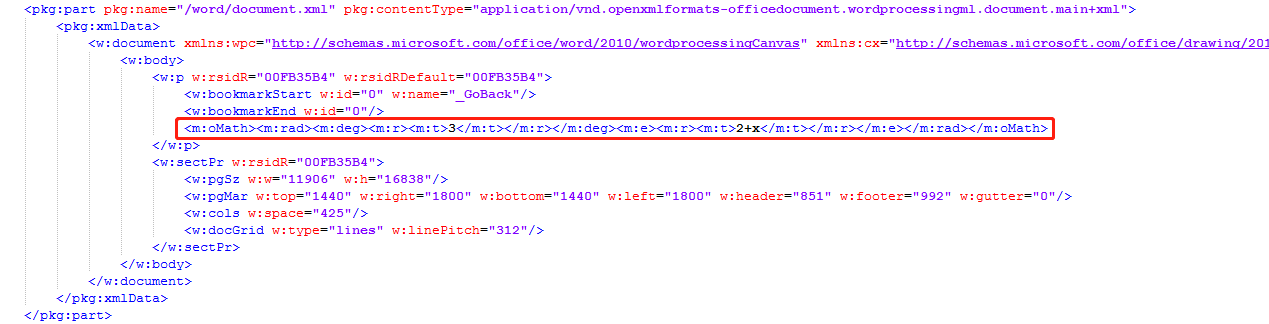## latex转word公式 java （latextoword,latex_word,latex2word,latex_omml）

latex_word

•     给大家分享一个我的原创作品：latex转为word公式（omml）工具 [java]
•     此工具主要用于将含有latex公式的文本下载成word时，将latex转换为word格式的公式
•     如果这不是你想要的你走吧，不要给我打赏

•     需要将html格式且带有latex公式的试题下载成word版的试卷
•     html转到word版并且要求一定的排版，还有一些可配置显示，这块也比较难弄，我的另一篇文章有分享
•     起初用了一些别人编写的转换工具，大多是先将latex转为mathml，再由mathml转为omml（word的公式），但其中有很多公式

转换不出来或转换出错，转换出错的再用另一种将latex转换为图片的形式，这样做到一个折中的方案，但在显示效果上图片相

对于word公式还是有很大差距的，另外图片的公式不能编辑，不是很理想，于是打算自己写一个转换工具

•     因此我的第一个原创作品出现了，给大家分享一下
 latex： mathml： omml： 一般转换步骤： $$\sqrt{2+x}$$ 2+x3[/itex] 32+x 我的工具： $$\sqrt{2+x}$$ 不需做中转，直接转omml 32+x

•   支持以 ,,, 为公式边界的latex代码的转换
$$\sqrt{2+x}$$   $\{\frac{3-a}{2+x}\}$    $3^(x+1)$    $$sum\limits_{i=1}^n$$
•   支持较复杂公式的转换
$$f(x)= \begin{cases}x,x>0 \\ 0,x=0 \\-x,x<0 \end{cases}$$
$$\left\{ \begin{array}{l}{x+y-1≥0} \\ {x-1≤0} \\ {ax-y+1≥0} \end{array} \right.$$
$$7+97+997+9997+\cdots\underbrace{99\cdots9}_{10个9}7$$
• 支持部分字体转换
$$37.5^\circ {\rm C}$$  $$str\bf{B}C^\circ$$

•   给诸位献上我的工具包：https://github.com/mao-yuwei/latex_word.git
•   将下载下来的 latex-word-1.0.x.jar导入本地maven库
•   依赖jar：jackson-databind-2.9.x.jar

mvn install:install-file -Dfile=(jar包的位置) -DgroupId=com.latextoword -DartifactId=latex-word -Dversion=1.0.x -Dpackaging=jar

•   pom添加依赖

        <dependency>
<groupId>com.latextoword</groupId>
<artifactId>latex-word</artifactId>
<version>1.0.x</version>
</dependency>
        <dependency>           <groupId>com.fasterxml.jackson.core</groupId>           <artifactId>jackson-databind</artifactId>           <version>2.9.3</version>        </dependency>
•   调用代码
    public static void main(String[] args) {
String latexStr="\$$\\sqrt{2+x}\$$";
String omml= Latex_Word.latexToWord(latexStr);
System.out.println(omml);
}
• 如果在调用之前你已经处理掉公式边界，用下面的方法
    public static void main(String[] args) {
String latexStr="\\sqrt{2+x}";
String omml= Latex_Word.latexToWordAlreadyClean(latexStr);
System.out.println(omml);
}
• 输出：无异常输出
<m:oMath><m:rad><m:deg><m:r><m:t>3</m:t></m:r></m:deg><m:e><m:r><m:t>2+x</m:t></m:r></m:e></m:rad></m:oMath>
• 输出：有异常
ERROR
• 有异常时日志会打印发生异常的位置（这种情况时，先检查latex公式有没有错误，如果没错请告诉我不能解析的公式，以便我及时修复，完善）
五月 16, 2019 2:39:43 下午 com.latextoword.Latex_Word latexToWordAlreadyClean

ERROR
• 验证公式转换的是否正确：
1.  >>新建word文档
2. >> 将word文档另存为 Word Xml 文档（*.xml）的格式
3. >>用NotePad++等工具以文本的形式打开另存后的.xml文件
4. >> 在<w:p..></w:p>标签之间粘贴进出转换后的omml公式，保存
5. >> 直接打开.xml文件就可以看见公式在word里面显示了此工具已经过大量公式测试，但应该还有不能转换的公式，如果有遇到敬请告知

公式展示字体相对大小这块没兼顾

在内容完整度上还是可以的

html试题试卷下载成word案例分享：https://www.cnblogs.com/maoyuwei/p/11637738.htmlposted on 2019-05-16 15:27  maoooooo  阅读(3029)  评论(12编辑  收藏  举报